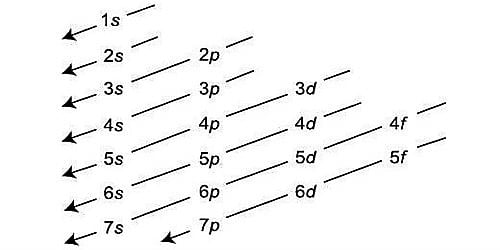# Does 2d orbital exist in an atom?

2d orbital can’t exist in an atom. We can explain it from its subsidiary quantum number and principal quantum number (n). The value ℓ gives the sub-shell or sub-level in a given principal energy shell to which an electron belongs. Because the quantum number n must be superior to angular momentum quantum number. In the 2nd energy level, electrons are placed only in the ‘s’ and ‘p’ sublevels, so there is no ‘d’ orbital.

For n=2, ℓ=0, 1

i.e. The second principal energy level consists of two sub-shell which is 2s (ℓ=0) and 2p (ℓ=1). So, 2d orbital can’t exist.

Thus, for n = 1, only l = 0 gives a valid wave function, for n = 2, you can only have l = 0, 1 (‘s’ and ‘p’), and so on. This form follows from the obligation that the wave function needs to be square integral.